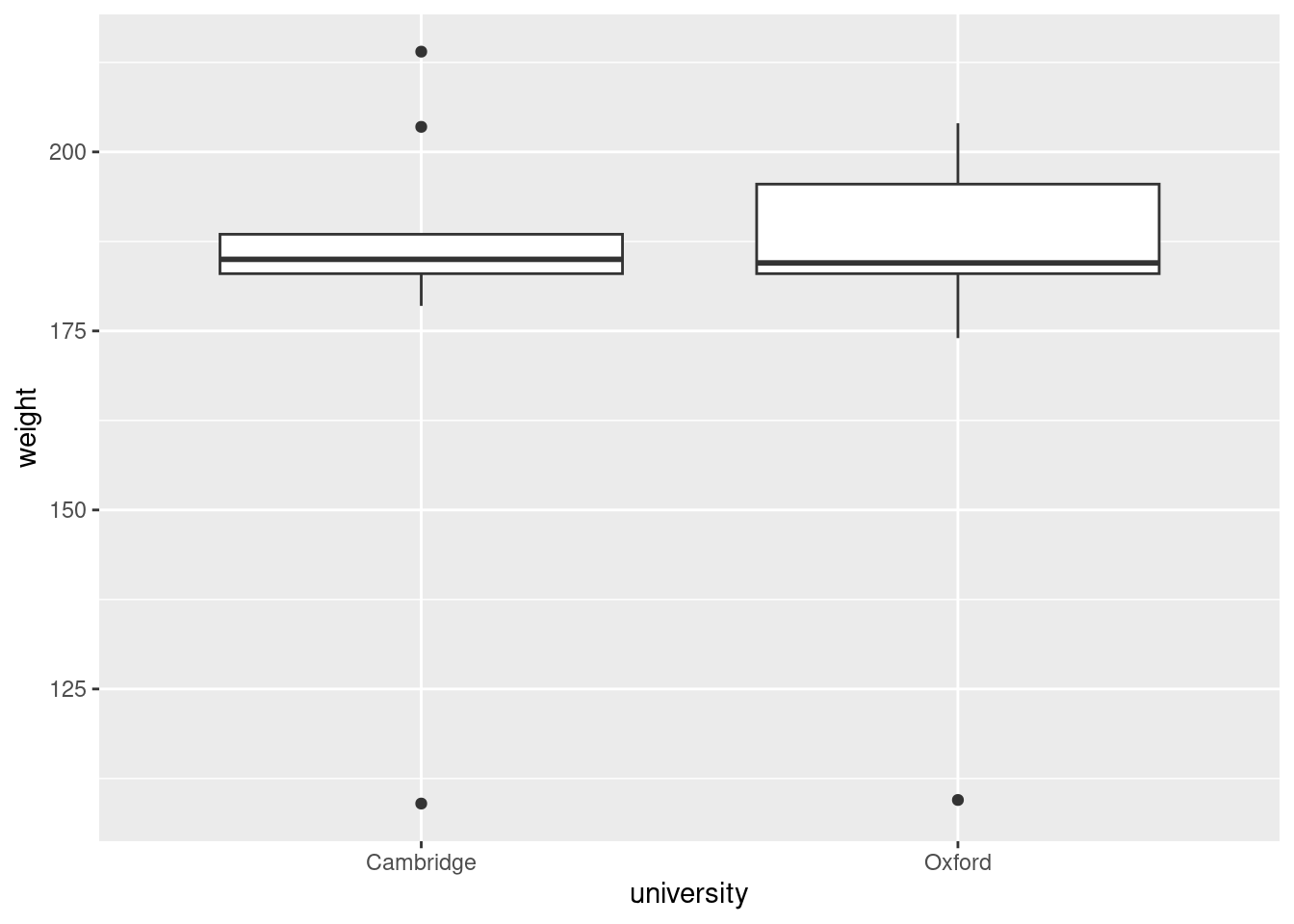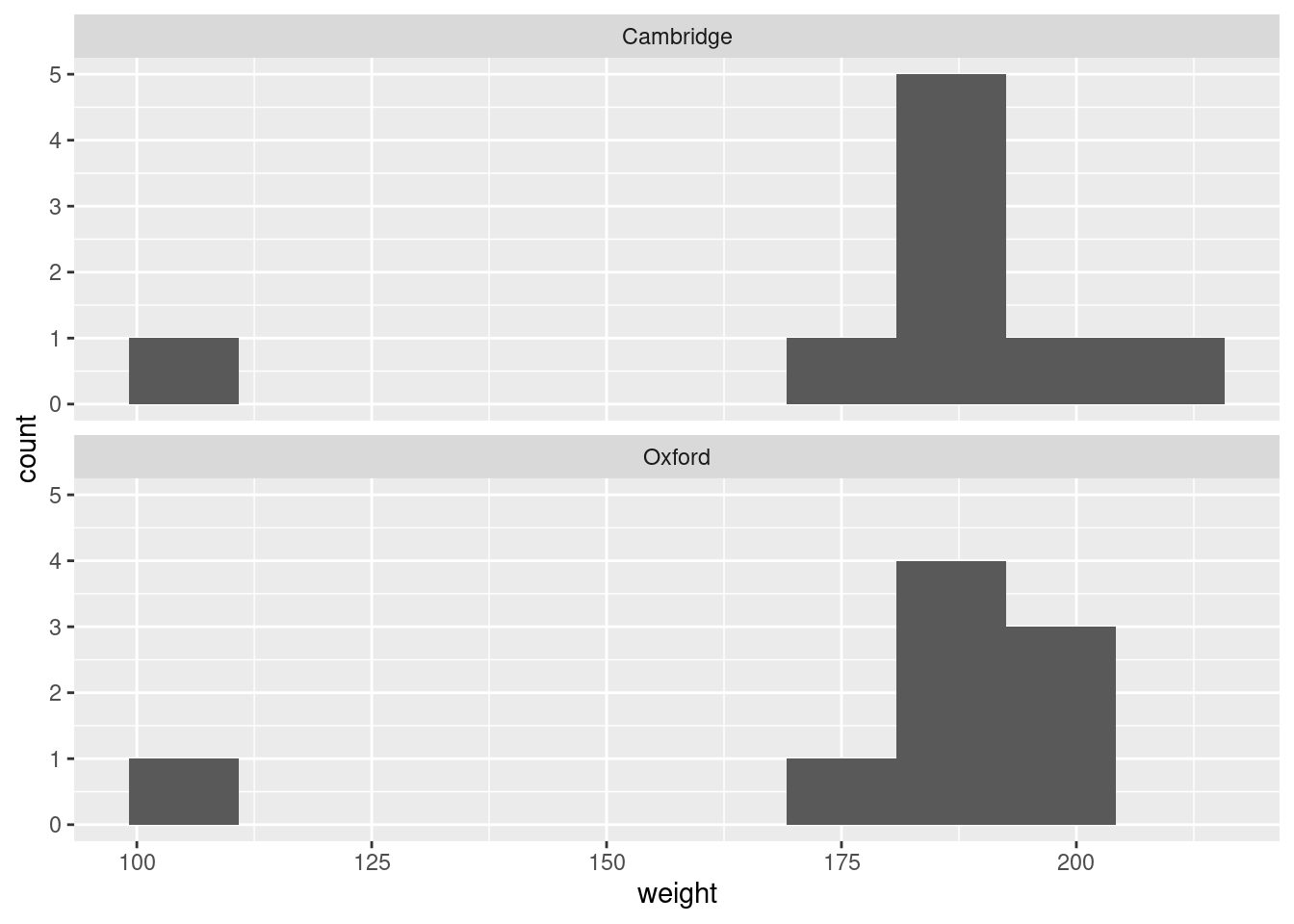# Worksheet 2

Published

September 8, 2023

Questions are below. My solutions are below all the question parts for a question; scroll down if you get stuck. There is extra discussion below that for some of the questions; you might find that interesting to read, maybe after tutorial. There is probably too much here for you to finish in an hour, but I encourage you to continue later with what you were unable to finish in tutorial.

For these worksheets, you will learn the most by spending a few minutes thinking about how you would answer each question before you look at my solution. There are no grades attached to these worksheets, so feel free to guess: it makes no difference at all how wrong your initial guess is!

# 1 The Boat Race

Each year, the Oxford and Cambridge University rowing teams race against each other on the River Thames in London (England). For the 1992 race, the weights (in pounds) of the participants on each team were recorded, and can be found in https://ritsokiguess.site/datafiles/boat_race.txt.

1. Take a look at the data file (in your web browser), and describe how the data values are separated one from the next.

2. Read the file into a dataframe and display at least some of it.

3. Make a suitable graph of your data.

4. Would you say, based on your plot, that the average or typical weights of the rowers on the two teams are similar or different? Explain briefly.

5. Each rowing team consists of eight rowers plus a cox, whose job is to keep the rowers in tempo. The cox does not row themselves. Which of the nine individuals in each team do you think is the cox? Explain briefly.

My solutions follow:

1. Take a look at the data file, and describe how the data values are separated one from the next.

Solution

Click on the URL, and see that the data values are separated by a single space. First comes the name of the university the rower comes from, then a single space, then the rower’s weight in pounds.

$$\blacksquare$$

1. Read the file into a dataframe and display at least some of it.

Solution

The data values are separated by a single space, so you need read_delim with a single space as the second input. My habit is to save the (often long) URL into a variable first:

my_url <- "https://ritsokiguess.site/datafiles/boat_race.txt"
rowers <- read_delim(my_url, " ")
Rows: 18 Columns: 2
── Column specification ────────────────────────────────────────────────────────
Delimiter: " "
chr (1): university
dbl (1): weight

ℹ Use spec() to retrieve the full column specification for this data.
ℹ Specify the column types or set show_col_types = FALSE to quiet this message.
rowers

You can check, by looking under the column heading, that the university names are text and the weights really are numbers (dbl means “double-precision decimal number”).

The alternative below works, but you have some extra work to do to explain why it works:

rowers0 <- read_delim(my_url)
Rows: 18 Columns: 2
── Column specification ────────────────────────────────────────────────────────
Delimiter: " "
chr (1): university
dbl (1): weight

ℹ Use spec() to retrieve the full column specification for this data.
ℹ Specify the column types or set show_col_types = FALSE to quiet this message.
rowers0

To get full credit for doing it this way (if it were on an assignment), you would need to draw the reader’s attention to this line:

Delimiter: " "

This means that read_delim guessed, by looking at the file before reading it in, that the data values seemed to be separated by single spaces. It had to guess, because you didn’t say what to look for.

This also works, but in this course is wrong:

rowers0 <- read.table(my_url, header = TRUE)
rowers0

If you do it this way, it reveals that you are not paying attention. In the course outline it says “I expect you to do things as they are done in this course”, and in the lecture it says that read_delim is how to read a file of this type. You may have learned things differently elsewhere, but I do not use read.table in this course at all (and indeed, not very many base R ideas).

If you know anything about rowing, you might be a bit suspicious about there being nine observations, since a rowing team usually has eight people. See the last part of the question.

$$\blacksquare$$

1. Make a suitable graph of your data.

Solution

First take a look at the type of variables you have: one categorical (the name of the university) and one quantitative (the weight of the rower). An appropriate graph for variables of this type is a side-by-side boxplot.

In ggplot the categorical variable is x because it goes horizontally, and the quantitative one is y (vertically):

ggplot(rowers, aes(x = university, y = weight)) + geom_boxplot()This is the best plot. About the only other useful plot at all is above and below histograms. To get those, make a histogram of weights, and then facet by university, displaying the results in one column:

ggplot(rowers, aes(x = weight)) + geom_histogram(bins = 10) +
facet_wrap(~university, ncol = 1)If you go this way, you will almost certainly have to experiment with the number of bins. One of the automatic bin choices is unlikely to help you here, since so many of the bins are empty. Also, the purpose of the plot is to compare the distributions, like the boxplot, so you really need the histograms to be above and below, with a common $$x$$-scale, not left and right with a common count scale.

$$\blacksquare$$

1. Would you say, based on your plot, that the average or typical weights of the rowers on the two teams are similar or different? Explain briefly.

Solution

The horizontal lines across the boxes on a boxplot are the medians of the distributions of weights. These medians are, I would say, very similar, especially given the amount of variability. (Have an opinion and defend it.)

From a boxplot, you cannot say anything about means, because they do not appear on a boxplot. With the kinds of distributions you have here, the mean is not a very sensible summary anyway, because of the outliers.

The use of the word “typical” in the question is meant to guide you towards a measure of centre, which might be mean, median, or even mode. A boxplot only shows you the median, which, as discussed, is a sensible measure of centre here anyway, so discuss that. (If your plot was the over-and-under histograms, you can try to figure out where, say, the medians are, or even use the mode. I care mostly about your thought process, not so much about the precise answer you get.)

$$\blacksquare$$

1. Each rowing team consists of eight rowers plus a cox, whose job is to keep the rowers in tempo. The cox does not row themselves. Which of the nine individuals in each team do you think is the cox? Explain briefly.

Solution

The obvious guess is “the low outlier”. But you also need to say something about why: if the cox does not row, this means that the other rowers are expending energy moving the cox as well as the boat. Thus it is an advantage to have a cox who is as light in weight as possible. Hence the low outlier is most probably the cox. (This was indeed the case.)

The cox on a rowing team has a similar role to the conductor in an orchestra, except that the musicians in an orchestra are not trying to move the conductor across the water as fast as possible!1

$$\blacksquare$$

# 2 Intensive Care Unit patients

The Intensive Care Unit (ICU) at a hospital is where incoming patients that need the most urgent treatment are admitted. When a patient is admitted, a large number of measurements are taken, to help the ICU doctor decide on an appropriate treatment. The variables of interest to us here are these two (there are actually many others, as you will see):

• sta: vital status (0 = lived, 1 = died)
• typ: type of admission (0 = elective, 1 = emergency)

The data for 200 patients were in http://www.medicine.mcgill.ca/epidemiology/Joseph/courses/EPIB-621/icudat.txt.

2. Read in and display (some of) the data.

3. Make a suitable graph of the two variables of interest. Make sure you consider what type of variable these are (which might not be the same as how they are recorded).

4. What do you learn from your graph, in the context of the data?

My solutions:

Solution

The columns are lined up. This is the most important thing, but you can also note that the values are separated by more than one space, so that read_delim will not work.

$$\blacksquare$$

1. Read in and display (some of) the data.

Use read_table for the aligned columns:

my_url <- "http://www.medicine.mcgill.ca/epidemiology/Joseph/courses/EPIB-621/icudat.txt"
icu <- read_table(my_url)

── Column specification ────────────────────────────────────────────────────────
cols(
.default = col_double()
)
ℹ Use spec() for the full column specifications.
icu